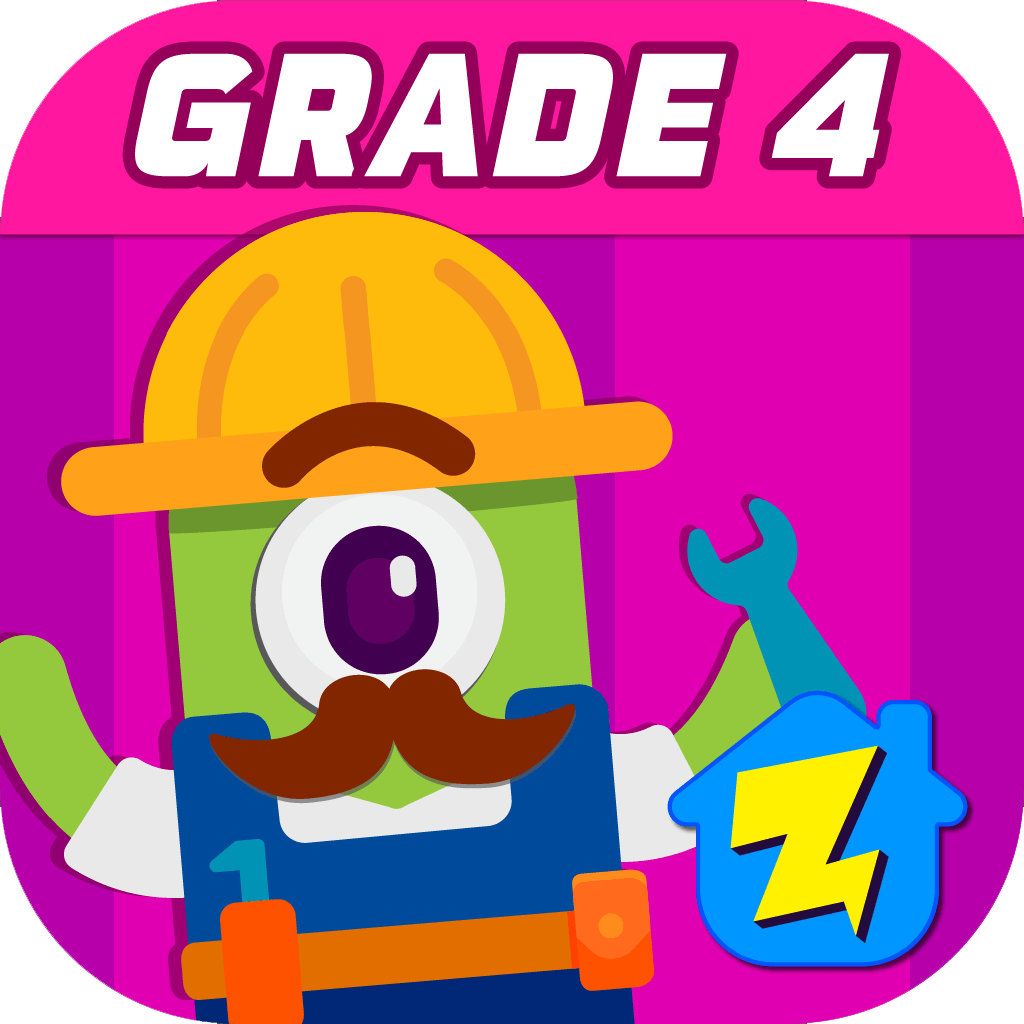## Welcome to Zapzapmath Grade 4 Home App### This app helps players understand the concept of factor pairs, number patterns, round numbers, equivalent fractions, decimals, angles, and classify shapes by their lines and angles.

Our 4th grade math games cover topics such as decimals, how to measure angles, and the relationships of place values. Most of the fourth grade students will find these games fascinating as these games help students have a thorough understanding of angle measurements, place values, and decimals via fun and interactive games.

If you are searching for material that is not only educational but also engaging, our games will work magic to help students embrace math topics. So go ahead and energize math learning with our math games below.

### Skills that can be learned

##### Multiplication and division

Relate multiplication and division to place values

##### Angles

Calculate angles using a protractor

Compare decimals

### Games Available In This Grade### How To Measure Angles : Angle Align

Recognize and measure angles### Number Place Values : Amazon Jones

Understand relative value of place values### Decimal Games : Decimal Shootout

Compare decimals to hundredths